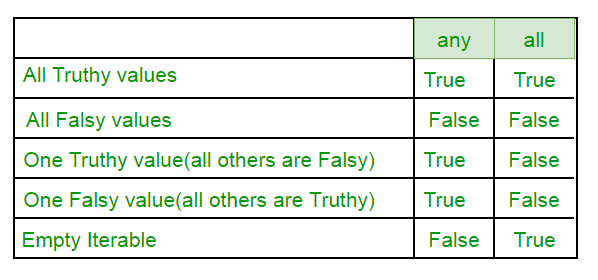Related Articles
Any All in Python
• Difficulty Level : Easy
• Last Updated : 25 Nov, 2020

Any and All are two built ins provided in python used for successive And/Or.

Any
Returns true if any of the items is True. It returns False if empty or all are false. Any can be thought of as a sequence of OR operations on the provided iterables.
It short circuit the execution i.e. stop the execution as soon as the result is known.

Syntax : any(list of iterables)

 `# Since all are false, false is returned``print` `(``any``([``False``, ``False``, ``False``, ``False``]))`` ` `# Here the method will short-circuit at the``# second item (True) and will return True.``print` `(``any``([``False``, ``True``, ``False``, ``False``]))`` ` `# Here the method will short-circuit at the``# first (True) and will return True.``print` `(``any``([``True``, ``False``, ``False``, ``False``]))`

Output :

```False
True
True
```

All
Returns true if all of the items are True (or if the iterable is empty). All can be thought of as a sequence of AND operations on the provided iterables. It also short circuit the execution i.e. stop the execution as soon as the result is known.

Syntax : all(list of iterables)

 `# Here all the iterables are True so all``# will return True and the same will be printed``print` `(``all``([``True``, ``True``, ``True``, ``True``]))`` ` `# Here the method will short-circuit at the ``# first item (False) and will return False.``print` `(``all``([``False``, ``True``, ``True``, ``False``]))`` ` `# This statement will return False, as no``# True is found in the iterables``print` `(``all``([``False``, ``False``, ``False``]))`

Output :

```True
False
False
```

Practical Examples

 `# This code explains how can we ``# use 'any' function on list ``list1 ``=` `[]``list2 ``=` `[]`` ` `# Index ranges from 1 to 10 to multiply``for` `i ``in` `range``(``1``,``11``):``    ``list1.append(``4``*``i) `` ` `# Index to access the list2 is from 0 to 9``for` `i ``in` `range``(``0``,``10``):``    ``list2.append(list1[i]``%``5``=``=``0``)`` ` `print``(``'See whether at least one number is divisible by 5 in list 1=>'``)``print``(``any``(list2))`

Output:

```See whether at least one number is divisible by 5 in list 1=>
True
```
 `# Illustration of 'all' function in python 3`` ` `# Take two lists ``list1``=``[]``list2``=``[]`` ` `# All numbers in list1 are in form: 4*i-3``for` `i  ``in` `range``(``1``,``21``):``    ``list1.append(``4``*``i``-``3``)`` ` `# list2 stores info of odd numbers in list1``for` `i ``in` `range``(``0``,``20``):``    ``list2.append(list1[i]``%``2``=``=``1``)`` ` `print``(``'See whether all numbers in list1 are odd =>'``)``print``(``all``(list2))`

Output:

```See whether all numbers in list1 are odd =>
True
```

Truth table :-This article is contributed by Mayank Rawat .If you like GeeksforGeeks and would like to contribute, you can also write an article using contribute.geeksforgeeks.org or mail your article to contribute@geeksforgeeks.org. See your article appearing on the GeeksforGeeks main page and help other Geeks.